# Texas Go Math Grade 6 Lesson 16.4 Answer Key Solving Volume Equations

Refer to our Texas Go Math Grade 6 Answer Key Pdf to score good marks in the exams. Test yourself by practicing the problems from Texas Go Math Grade 6 Lesson 16.4 Answer Key Solving Volume Equations.

## Texas Go Math Grade 6 Lesson 16.4 Answer Key Solving Volume Equations

Example 1

A rectangular swimming pool is 25 meters long and 17$$\frac{1}{2}$$ meters wide. It has an average depth of 1$$\frac{1}{2}$$ meters. What is the volume of the pool?Label the rectangular prism to represent the pool.
l = 25 meters; w = 17$$\frac{1}{2}$$ meters; h = 1$$\frac{1}{2}$$ meters
Use the formula to write an equation.
v = lwh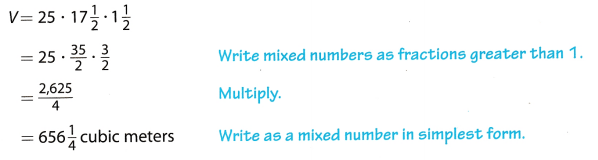Question 1.
Miguel has a toolbox that measures 18$$\frac{1}{2}$$ inches by 12$$\frac{1}{2}$$ inches by 4 inches. What is the volume of the toolbox?
V = _________ cubic inches
Determine the volume of the toolbox.
V = 18$$\frac{1}{2}$$ ∙ 12$$\frac{1}{2}$$ ∙ 4 substitute for the given values
V = $$\frac{37}{2}$$ ∙ $$\frac{25}{2}$$ ∙ 4 write mixed numbers as fractions
V = $$\frac{3,700}{4}$$ simplify
V = 925 cubic inches volume of the toolbox
The volume of the toolbox is 925 cubic inches

Example 2

Samuel has an ant farm with a volume of 375 cubic inches. The width of the ant farm is 2.5 inches and the length is 15 inches. What is the height of Samuel’s ant farm?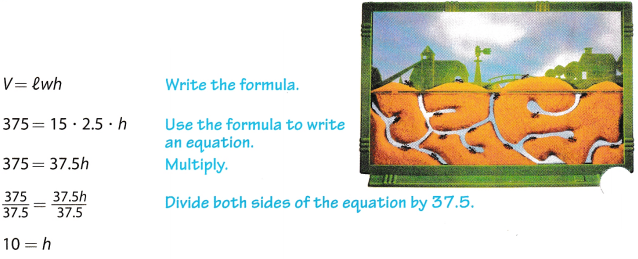The height of the ant farm is 10 inches.

Reflect

Question 2.
Communicate Mathematical Ideas Explain how you would find the solution to Example 2 using the formula V = 8h.
Here B = l × w can be evaluated and then the given volume can be divided by B to evaluate h.

Question 3.
Find the height of this shape, which has a volume of $$\frac{15}{16}$$ cubic feet.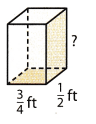Data:
l = $$\frac{3}{4}$$ = 0.75
w = $$\frac{1}{2}$$ = 0.5
Volume = $$\frac{15}{16}$$ = 0.9375
Write equation of volume of a rectangular prism:
VoLume = l × w × h
Solve for h:
h = $$\frac{\text { Volume }}{l \times w}$$
Substitute values:
h = $$\frac{0.9375}{0.75 \times 0.5}$$
Evaluate:
h = 2.5
Height of the given rectangular prism is equal to 2.5 = 2$$\frac{1}{2}$$ feet.

Example 3

The classroom aquarium holds 30 gallons of water. It is 0.8 feet wide and has a height of 2 feet. Find the length of the aquarium.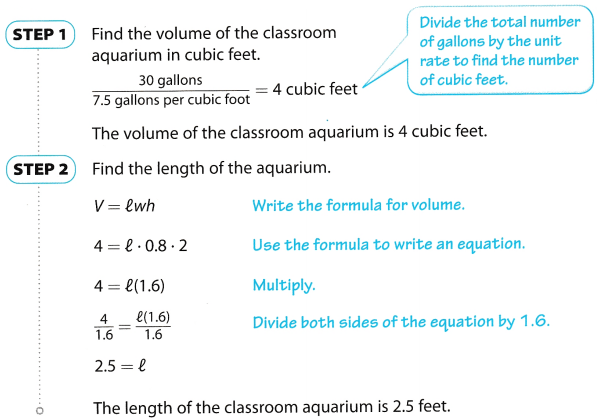Reflect

Question 4.
Persevere in Problem Solving Flow much does the water in the classroom aquarium weigh? Explain.
The volume of the classroom aquarium is 4 cubic feet and the equivalent of 1 cubic feet is approximately 62.43 pounds. Therefore, multiply the volume by the estimated weight.
62.43 × 4 = 249.72 pounds
The water in the aquarium weighs about 249.72 pounds.

Question 5.
An aquarium holds 33.75 gallons of water. It has a length of 2 feet and a height of 1.5 feet. What is the volume of the aquarium? What is the width of the aquarium? Explain.
Determine the volume and width of the aquarium.
V = $$\frac{33.75}{7.5}$$ volume of the aquarium in cubic feet
V = 4.5 cubic feet
V = lwh formula for the volume of the aquarium
4.5 = 2 ∙ w ∙ 1.5 substitute for the given values
4.5 = 3w simplify
$$\frac{4.5}{3}=\frac{3 w}{3}$$ divide both sides of the equation by 3
1.5 = w width of the aquarium
The volume of the aquarium is 4.5 cubic feet and the width is 1.5 feet.

Question 1.
Find the volume of this rectangular prism. (Example 1)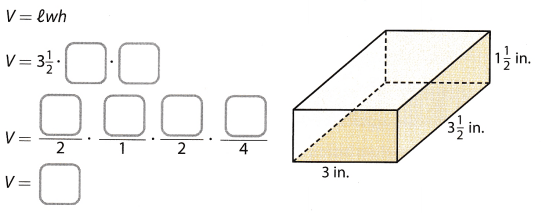The volume of the rectangular prism is _________ cubic inches.
Determine the volume.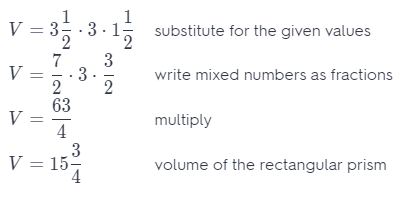The volume of the rectangular prism is 15$$\frac{3}{4}$$ cubic inches.

Question 2.
Write an equation to find the width of the rectangular prism. Show your work. (Example 2)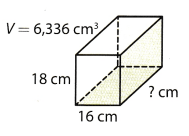Determine the width of the rectangular prism
V = lwh formula for the volume of the rectangular prism
w = $$\frac{V}{l h}$$ equation for the width of the prism
w = $$\frac{6,336 \mathrm{~cm}^{3}}{16 \mathrm{~cm} \cdot 18 \mathrm{~cm}}$$ substitute for the given values
w = $$\frac{6,336 \mathrm{~cm}^{3}}{288 \mathrm{~cm}^{2}}$$ simplify
w = 22 cm width of the rectangular prism
The width of the rectangular prism is 22 cm.

Question 3.
One red clay brick weighs 5.76 pounds. The brick is 8 inches long and 2 inches wide. If the clay weighs 0.08 pounds per cubic inch, what is the volume of the brick? Write an equation to find the height of the brick. Show your work. (Example 3)
Determine the volume and height of the brick.
V = $$\frac{5.76}{0.08}$$ volume of the brick in cubic inches
V = 72 cubic inches
V = lwh formula for the volume of the brick
72 = 8 ∙ 2.25 ∙ h substitute for the given values
72 = 18h simplify
$$\frac{72}{18}=\frac{18 h}{18}$$ divide both sides of the equation by 18
4 inches = h height of the brick
The bricks is 4 inches in height.

Essential Question Check-In

Question 4.
How do you solve problems about volume of right rectangular prisms?
Solving problems involving the volume of right rectangular prisms, identify the length, width, and height of the rectangular prism then get its product.

Question 5.
Jala has an aquarium in the shape of a rectangular prism with the dimensions shown. What is the height of the aquarium?Height = ___________
Solution to this example is given beLow
l = 24.25 inches; w = 12.5 inches; h = ? ; V = 3758.75 in3
V = lwh Write the formula.
3758.75 = 24.25 × 12.5 × h Use the formula to write an equation
3758.75 = (303.125)h Multiply
$$\frac{3758.75}{303.125}=\frac{303.125 h}{303.125}$$ Divide both sides of the equation by 303125.
12.4 = h
Height of the given aquarium is 12.4 inches

Question 6.
Find the volume of a juice box that is 3 in. by 1$$\frac{1}{2}$$ in. by 4 in.
Volume = ______________
Determine the voLume of the juice box.
V = 3 ∙ 1.5 ∙ 4 substitute for the given values
V = 18 in3 volume of the juice box
The volume of the juice box is 18 cubic inches.

Question 7.
Find the width of a cereal box that has a volume of 3,600 cm3 and is 20 cm long and 30 cm high.
Width = ____________
Determine the width of the box.
V = lwh formula for the volume of box
3, 600 = 20 ∙ w ∙ 30 substitute for the given values
3,600 = 600w simplify
$$\frac{3,600}{600}=\frac{600 w}{600}$$ divide both sides of the equation by 600
6 cm = w width of the cereal box
The width of the cereal box is 6 cm.

Question 8.
Bill has a box of markers that has a base of 8 cm by 20 cm and a height of 6 cm. Martin’s pencil box has a height of 4 cm and a base that is 15 cm by 16 cm. Bill says his marker box has the same volume as Martin’s pencil box. Is Bill right? Explain.
Determine the volume of both objects then compare.
V = 8 ∙ 20 ∙ 6 substitute for the dimensions of the box of markers
V = 960 cm3 volume of the box of markers
V = 4 ∙ 15 ∙ 16 substitute for the dimensions of the pencil box
V = 960 cm3 volume of the pencil box
Yes, Bill is right with his conjecture because the volume of the box of marker and pencil box are both the same.

Question 9.
Physical Science A small bar of gold measures 40 mm by 25 mm by 2 mm. One cubic millimeter of gold weighs about 0.0005 ounces. Find the volume in cubic millimeters and the weight in ounces of this small bar of gold.
Data:
Density = 0.0005
l = 40
w = 25
h = 2
Write equation of volume of a rectangular prism:
VoLume = l × w × h
Substitute vaLues:
Volume = 40 × 25 × 2
Evaluate:
Volume = 2000
Volume of the bLock is 2000 cubic millimeters.

Write equation of density of a substance:
Density = $$\frac{\text { mass }}{\text { Volume }}$$
Solve for mass:
mass = Density × Volume
Substitute values:
mass = 0.0005 × 2000
Evaluate:
mass = 1
Mass of this block is 1 ounce.

Question 10.
History The average stone on the lowest level of the Great Pyramid in Egypt was a rectangular prism 5 feet long by 5 feet high by 6 feet deep and weighed 15 tons. What was the volume of the average stone? How much did one cubic foot of this stone weigh?
Data:
mass = 15
l = 5
w = 5
h = 6
Write equation of volume of a rectangular prism:
Volume = l × w × h
Substitute values:
VoLume = 5 × 5 × 6
Evaluate:
Volume = 150
Volume of the block is 150 cubic feet.

Write equation of density of a substance:
Density = $$\frac{\text { mass }}{\text { Volume }}$$
Substitute values:
Density = $$\frac{15}{150}$$
Evaluate:
Density = 0.1
Density of this block is 0.1 ton per cubic feet.

Question 11.
A freshwater fish is healthiest when there is at least one gallon of water for every inch of its body length. Roshel wants to put a goldfish that is about 2$$\frac{1}{2}$$ inches long in her tank. Roshel’s tank is 7 inches long, 5 inches wide, and 7 inches high. The volume of 1 gallon of water is about 231 cubic inches.
a. How many gallons of water would Roshel need for the fish?
a. According to the given information, the amount of water needed by the goldfish is 2$$\frac{1}{2}$$ × 1 = 2$$\frac{1}{2}$$ = 2.5 gallons of water. This is equal to 2.5 × 231 = 577.5 cubic inches.

b. What is the volume of Roshel’s tank?
Volume of the tank is 7 × 5 × 7 = 245 cubic inches.

c. Is her fish tank large enough for the fish? Explain.
Since the volume of water required is 577.5 > 245, her tank is not large enough to store the amount of water required by the fish.

Question 12.
A box of crackers is a rectangular box with the dimensions shown. The box is one-fourth full. What is the volume of crackers in the box? _______________________________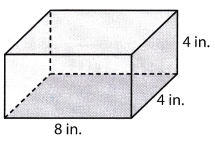Determine the volume of the crackers in the box.
V = 8 ∙ 4 ∙ 4 substitute for the given values
V = 128 in3 volume of the box
V = $$\frac{128}{4}$$ divide the volume by 4
V = 32 in3 volume of the crackers in the box
The volume of the crackers in the box is 32 cubic inches.

H.O.T. Focus on Higher Order Thinking

Question 13.
Multistep Larry has a clay brick that is 7 inches long, 3.5 inches wide, and 1.75 inches thick, the same size as the gold stored in Ft. Knox in the form of gold bars. Find the volume of this brick. If the weight of the clay in the brick is 0.1 pound per cubic inch and the weight of the gold is 0.7 pounds per cubic inch, find the weight of the brick and the gold bar. Round all answers the nearest tenth.
Volume of the brick or bar = __________ cubic inches
Weight of the brick = __________ pounds
Weight of the gold bar = __________ pounds
VoLume of the brick or bar is 7 × 3.5 × 1.75 = 42.875 cubic inches.
Weight of the brick in pounds is the product of its given density and its volume, therefore its weight is 42.875 × 0.1 = 4.2875 pounds.
Weight of the bar in pounds is the product of its given density and its voLume, therefore its weight is 42.875 × 0.7 = 30.0125 pounds.

Question 14.
Represent Real-World Problems Luisa’s toaster oven, which is in the shape of a rectangular prism, has a base that is 55 cm long by 40 cm wide. It is 30 cm high. Luisa wants to buy a different oven with the same volume but a smaller length, so it will fit better on her kitchen counter. What is a possible set of dimensions for this different oven?
VoLume of this toaster is 55 × 40 × 30 = 66000 cubic centimeters.
The volume of the new toaster must be same but with different dimensions provided that l < 55 centimeters, therefore:
Volume = 66000 = 48 × 42 × 32.74
Note that the length of the new toaster is less than 55 but to maintain the same volume, the width and height of the toaster has increased.

Question 15.
Multiple Representations Use the formula V = Bh to write a different version of this formula that you could use to find the area of the base B of a rectangular prism if you know the height h and the volume V. Explain what you did to find this equation.
Write equation of volume of a rectangular prism in terms of base Area B:
Volume = B × h
Divide both sides of this equation with h to solve for B: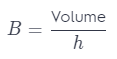This is the equation of the same formula for B.

Question 16.
Communicate Mathematical Ideas Explain how you could find the volume of a cube that has an edge of e.
Determine the volume of cube.
V = e ∙ e ∙ e multiply the edge of the cube by itself three times
V = e3 volume of the cube
Tne volume of the cube is e3.

Question 17.
Justify Reasoning Mariel says that a jewelry box that is 3 inches high, 4$$\frac{1}{2}$$ inches wide, and 5 inches long has a volume of 67$$\frac{1}{2}$$ inches. Katy says that answer is not quite correct. What is the error in Mariel’s answer?